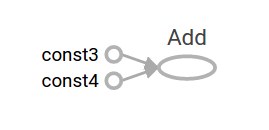# 底层 API 编程介绍

• 管理你的 TensorFlow 程序（一张 TensorFlow 计算图，`tf.Graph`）以及 TensorFlow 运行时（一个 TensorFlow 会话，`tf.Session`），而不是依赖 Estimators 来管理它们
• 使用 `tf.Session` 运行 TensorFlow 操作
• 在底层环境中使用高级的组件（datasets, layers, 以及 特征列

• 使用低级 TensorFlow 的操作能帮你更加切中肯綮地进行实验和 debug
* 在使用高层次 API 的时候，你能够知道其内部是如何运作的

## 配置

•   会使用 Python 进行编程
• 关于数组的一些知识
• 最好了解一些机器学习的内容

``````from __future__ import absolute_import
from __future__ import division
from __future__ import print_function

import numpy as np
import tensorflow as tf
``````

## 张量值

TensorFlow 核心的数据单元是张量（Tensor），一个张量由一个基本数据类型的多维数组来表示。张量的指的是它的维度数量，它的形状是一个整数元组，指定了各个维度的数组长度。下面一些张量值的例子：

``````3. # 秩为 0 的张量，形为 [] 的标量
[1., 2., 3.] # 秩为 1 的张量，形为  的向量
[[1., 2., 3.], [4., 5., 6.]] # 秩为 2 的张量，形为 [2, 3] 的矩阵
[[[1., 2., 3.]], [[7., 8., 9.]]] # 秩为 3 的张量，形为 [2, 1, 3]
``````

TensorFlow 使用 numpy 数组来表示张量的

## TensorFlow 核心 API 实战

### 计算图

``````a = tf.constant(3.0, dtype=tf.float32)
b = tf.constant(4.0) # 隐式的创建一个浮点类型
total = a + b
print(a)
print(b)
print(total)
``````

`print` 语句的结果如下：

``````Tensor("Const:0", shape=(), dtype=float32)
Tensor("Const_1:0", shape=(), dtype=float32)
``````

### TensorBoard

TensorFlow 提供了一个名为 TensorBoard 的工具。TensorBoard 的功能之一就是可视化一张计算图，几个简单的命令就能实现它。

``````writer = tf.summary.FileWriter('.')
``````

``````events.out.tfevents.{timestamp}.{hostname}
``````

``````tensorboard --logdir .
``````TensorBoard: 图形可视化

### 会话

`tf.Session`

``````sess = tf.Session()
print(sess.run(total))
``````

``````7.0
``````

``````print(sess.run({'ab':(a, b), 'total':total}))
``````

``````{'total': 7.0, 'ab': (3.0, 4.0)}
``````

``````vec = tf.random_uniform(shape=(3,))
out1 = vec + 1
out2 = vec + 2
print(sess.run(vec))
print(sess.run(vec))
print(sess.run((out1, out2)))
``````

``````[ 0.52917576  0.64076328  0.68353939]
[ 0.66192627  0.89126778  0.06254101]
(
array([ 1.88408756,  1.87149239,  1.84057522], dtype=float32),
array([ 2.88408756,  2.87149239,  2.84057522], dtype=float32)
)
``````

### 赋值

``````x = tf.placeholder(tf.float32)
y = tf.placeholder(tf.float32)
z = x + y
``````

`tf.Session.run`

``````print(sess.run(z, feed_dict={x: 3, y: 4.5}))
print(sess.run(z, feed_dict={x: [1, 3], y: [2, 4]}))
``````

``````7.5
[ 3.  7.]
``````

## 数据集

`tf.data`

`tf.data.Iterator.get_next`

`tf.data.Dataset.make_one_shot_iterator`

``````my_data = [
[0, 1,],
[2, 3,],
[4, 5,],
[6, 7,],
]
slices = tf.data.Dataset.from_tensor_slices(my_data)
next_item = slices.make_one_shot_iterator().get_next()
``````

`tf.errors.OutOfRangeError`

``````while True:
try:
print(sess.run(next_item))
except tf.errors.OutOfRangeError:
break
``````

``````r = tf.random_normal([10,3])
dataset = tf.data.Dataset.from_tensor_slices(r)
iterator = dataset.make_initializable_iterator()
next_row = iterator.get_next()

sess.run(iterator.initializer)
while True:
try:
print(sess.run(next_row))
except tf.errors.OutOfRangeError:
break
``````

## 网络层

`tf.layers`

### 创建网络层

`tf.layers.Dense`

``````x = tf.placeholder(tf.float32, shape=[None, 3])
linear_model = tf.layers.Dense(units=1)
y = linear_model(x)
``````

### 初始化网络层

``````init = tf.global_variables_initializer()
sess.run(init)
``````

### 执行网络层

``````print(sess.run(y, {x: [[1, 2, 3],[4, 5, 6]]}))
``````

``````[[-3.41378999]
[-9.14999008]]
``````

### 网络层函数快捷键

`tf.layers.dense`

``````x = tf.placeholder(tf.float32, shape=[None, 3])
y = tf.layers.dense(x, units=1)

init = tf.global_variables_initializer()
sess.run(init)

print(sess.run(y, {x: [[1, 2, 3], [4, 5, 6]]}))
``````

`tf.layers.Layer`

## 特征列

`tf.feature_column.indicator_column`

``````features = {
'sales' : [, , , ],
'department': ['sports', 'sports', 'gardening', 'gardening']}

department_column = tf.feature_column.categorical_column_with_vocabulary_list(
'department', ['sports', 'gardening'])
department_column = tf.feature_column.indicator_column(department_column)

columns = [
tf.feature_column.numeric_column('sales'),
department_column
]

inputs = tf.feature_column.input_layer(features, columns)
``````

`tf.tables_initializer`

``````var_init = tf.global_variables_initializer()
table_init = tf.tables_initializer()
sess = tf.Session()
sess.run((var_init, table_init))
``````

``````print(sess.run(inputs))
``````

``````[[  1.   0.   5.]
[  1.   0.  10.]
[  0.   1.   8.]
[  0.   1.   9.]]
``````

## 训练

### 定义数据

``````x = tf.constant([, , , ], dtype=tf.float32)
y_true = tf.constant([, [-1], [-2], [-3]], dtype=tf.float32)
``````

### 定义模型

``````linear_model = tf.layers.Dense(units=1)

y_pred = linear_model(x)
``````

``````sess = tf.Session()
init = tf.global_variables_initializer()
sess.run(init)

print(sess.run(y_pred))
``````

``````[[ 0.02631879]
[ 0.05263758]
[ 0.07895637]
[ 0.10527515]]
``````

### 损失函数

`tf.losses`

``````loss = tf.losses.mean_squared_error(labels=y_true, predictions=y_pred)

print(sess.run(loss))
``````

``````2.23962
``````

### 训练

`tf.train.GradientDescentOptimizer`

``````optimizer = tf.train.GradientDescentOptimizer(0.01)
train = optimizer.minimize(loss)
``````

``````for i in range(100):
_, loss_value = sess.run((train, loss))
print(loss_value)
``````

``````1.35659
1.00412
0.759167
0.588829
0.470264
0.387626
0.329918
0.289511
0.261112
0.241046
...
``````

### 完整程序

``````x = tf.constant([, , , ], dtype=tf.float32)
y_true = tf.constant([, [-1], [-2], [-3]], dtype=tf.float32)

linear_model = tf.layers.Dense(units=1)

y_pred = linear_model(x)
loss = tf.losses.mean_squared_error(labels=y_true, predictions=y_pred)

train = optimizer.minimize(loss)

init = tf.global_variables_initializer()

sess = tf.Session()
sess.run(init)
for i in range(100):
_, loss_value = sess.run((train, loss))
print(loss_value)

print(sess.run(y_pred))
``````